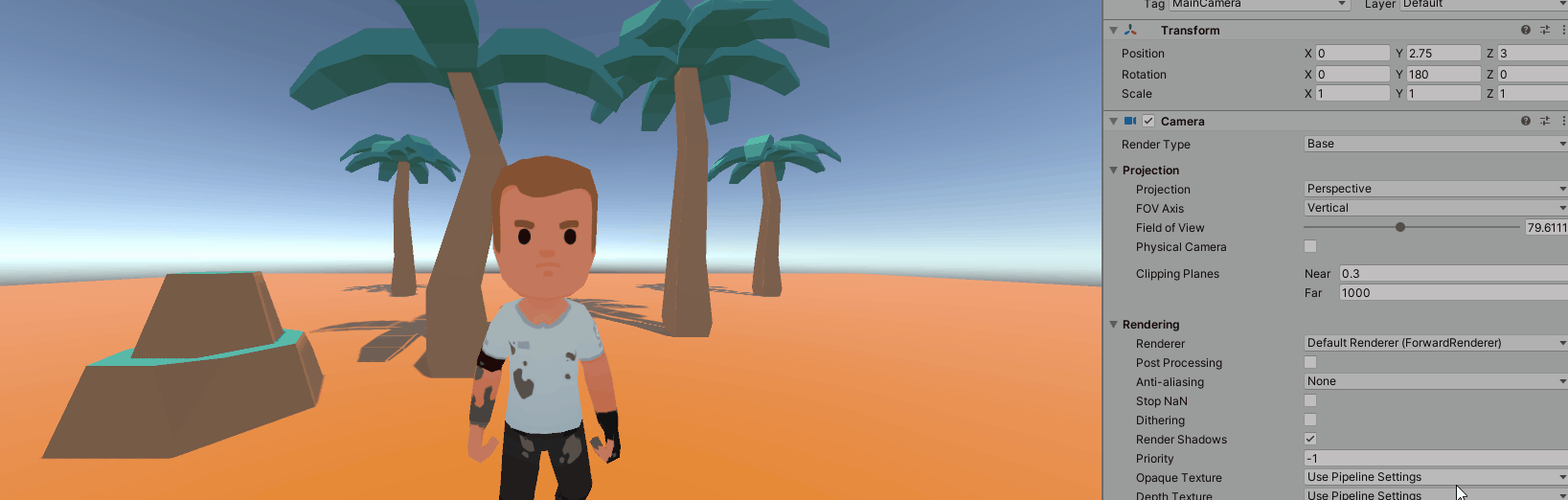Taking inspiration from movies is a great idea when making a video game. It can really help give a personal touch to many scenes and set your game apart from other creations.

In this article, I’ll be showing you how to implement the Dolly Zoom Effect in Unity. If you need a refresher, I definitely recommend watching this great 4 minute video!

The principle behind the Dolly Zoom is simple: when moving the camera closer, or further, from our subject, the field of view of the camera is adjusted so that the perceived distance between the camera and the subject stays the same. By doing this, the background becomes distorted while the subject stays the same. This can give a very trippy effect, as shown in the gif above, but it can also be more subtle.

While this is a powerful visual effect, the mathematical formula to replicate this effect is actually fairly simple:

By using this formula, we now know how to adjust the Field of View (FOV in the equation) when our distance changes, depending on a desired (constant) width of the scene, and this is exactly what we do in the code below: if the z-pos of the camera changes, the Field of View angle of the camera will be adjusted. But also, if the Field of View is adjusted in the editor, then the z-pos will be also modified accordingly. In the code I’m using local position, and my subject is my player so I’ve wrapped the model of the player and the camera in a single parent.

BE CAREFUL: the fieldOfView property of the camera uses degrees, while our formula uses radians. This is a common problem to remember, we’ll just need to multiply the constants Mathf.Rad2Deg and Mathf.Deg2Rad when appropriate.

``````public class DollyZoom : MonoBehaviour
{
// based around the formula:
// distance = width / (2 * tan(1/2 * FOV)))

public float width = 5f;
public float distance = 0f;
public float fieldOfView = 60f;

private Camera _camera;

private void Start()
{
_camera = transform.GetComponent<Camera>();
distance = transform.localPosition.z;

// set initial FOV value for camera
fieldOfView = Mathf.Rad2Deg * Mathf.Atan(width / (2 * distance)) * 2;
_camera.fieldOfView = fieldOfView;

}

private void Update()
{
if (_camera.fieldOfView != fieldOfView)
{
fieldOfView = _camera.fieldOfView;
distance = width / (2 * Mathf.Tan(0.5f *  Mathf.Deg2Rad * fieldOfView));

Vector3 localPos = transform.localPosition;
localPos.z = distance;
transform.localPosition = localPos;

} else if (distance != transform.localPosition.z)
{
distance = transform.localPosition.z;
fieldOfView = Mathf.Rad2Deg * Mathf.Atan(width / (2 * distance)) * 2;
_camera.fieldOfView = fieldOfView;

}
}

}
``````

Let’s see how it looks with a simple scene:The Effect looks extremely similar to what we can see in movies. Good Job!

It’s also interesting to note that when the Field of View becomes extremely small, or the distance becomes large, our camera tends to act like an orthographic camera and puts everything on the same level.